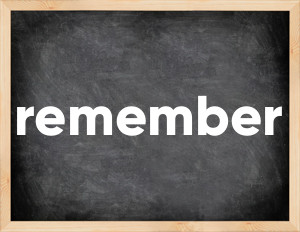# Remember past tenseThe English verb 'remember' is pronounced as [rɪˈmembə].
Related to: regular verbs.
3 forms of verb remember: Infinitive (remember), Past Simple - (remembered), Past Participle - (remembered).

## Here are the past tense forms of the verb remember

👉 Forms of verb remember in future and past simple and past participle.
❓ What is the past tense of remember.

## Remember: Past, Present, and Participle Forms

Base Form Past Simple Past Participle
remember [rɪˈmembə]

remembered [rɪˈmembəd]

remembered [rɪˈmembəd]

## What are the 2nd and 3rd forms of the verb remember?

🎓 What are the past simple, future simple, present perfect, past perfect, and future perfect forms of the base form (infinitive) 'remember'?

### Learn the three forms of the English verb 'remember'

• the first form (V1) is 'remember' used in present simple and future simple tenses.
• the second form (V2) is 'remembered' used in past simple tense.
• the third form (V3) is 'remembered' used in present perfect and past perfect tenses.

## What are the past tense and past participle of remember?

The past tense and past participle of remember are: remember in past simple is remembered, and past participle is remembered.

### What is the past tense of remember?

The past tense of the verb "remember" is "remembered", and the past participle is "remembered".

### Verb Tenses

Past simple — remember in past simple remembered (V2).
Future simple — remember in future simple is remember (will + V1).
Present Perfect — remember in present perfect tense is remembered (have/has + V3).
Past Perfect — remember in past perfect tense is remembered (had + V3).

### remember regular or irregular verb?

👉 Is 'remember' a regular or irregular verb? The verb 'remember' is regular verb.

## Examples of Verb remember in Sentences

•   This list is too long to remember (Present Simple)
•   She remembered something about that place (Past Simple)
•   Dr. Ian hasn't remembered it yet (Present Perfect)
•   I can't remember his name (Present Simple)
•   I think I remember this movie (Present Simple)
•   I remember him saying that before (Present Simple)
•   You remembered my favorite band is the Cure (Past Simple)
•   Hope you remembered the extra cheese (Past Simple)
•   He is a writer that people will remember (Future Simple)
•   I will always remember you (Future Simple)

Along with remember, words are popular raise and bind.

Verbs by letter: , , , , , , , , , , , , , , , , , , , , , , , , .